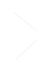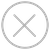TensorSpace.js
Getting StartBasic ConceptsTensorSpace ConverterModel PreprocessingModelsLayersMerge FunctionInstall TensorSpace Converter
Install the tensorspacejs pip package:
``\$ pip install tensorspacejs``
If tensorspacejs is installed successfully, you can check the TensorSpace-Converter version by using the command:
``\$ tensorspacejs_converter -v``
Then init TensorSpace Converter (important step):
``\$ tensorspacejs_converter -init``
wb_sunnyNote:
TensorSpace-Converter requires to run under Python 3.6, Node 11.3+, NPM 6.5+. If you have other pre-installed Python version in your local environment, we suggest you to create a new fresh virtual environment. For example, the conda commands is like:
``````\$ conda create -n envname python=3.6
\$ source activate envname
\$ pip install tensorspacejs``````